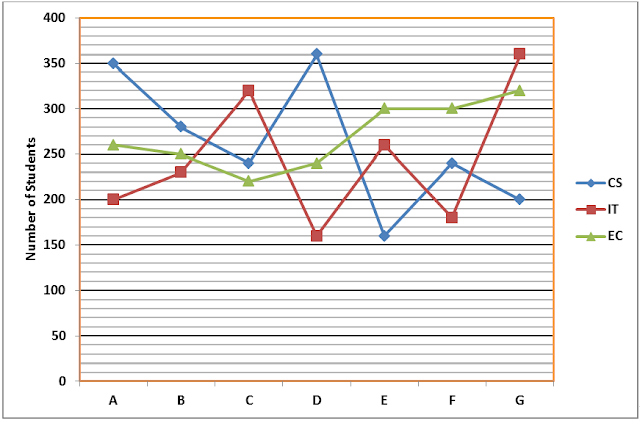Quantitative Practice Problems for IBPS Clerk (Set - 1)

Quantitative Practice Problems for IBPS Clerk (Set - 1)
1. 12 buckets of water fill a tank when the capacity of each bucket is 13.5 litres. How many buckets will be needed to fill the same tank, if the capacity of each bucket is 9 litres?
A) 9
B) 10
C) 11
D) 12
E) 18
2. The compound interest earned by Suresh on a certain amount at the end of two years at the rate of 8 p.c.p.a was Rs. 1,414.4. What was the total amount that Suresh got back at the end of two years in the form of principal plus interest earned?
A) Rs. 9,414.4
B) Rs. 9,914.4
C) Rs. 9,014.4
D) Rs. 8,914.4
E) None of these
3. Mr. Shah’s monthly income is Rs. 54550. In an entire year, he spends 32% of his annual salaries on groceries, he spend 12% on repairs and 10% he pays to his servant. If half of the remaining amount he invests in fixed deposits, what is the amount invested by him in fixed deposits?
A) Rs. 150558
B) Rs. 155240
C) Rs. 152610
D) Rs. 158789
E) Rs. 154336
4. Five years ago, the average age of Rimi’s age at that time was 12 years. Three years from now if the respective ratio between Rimi’s age and Ruhi’s age that time will be 5:3, what is Rimi’s present age?
A) 17 years
B) 33 years
C) 27 years
D) 22 years
E) 25 years
5. Two containers contain spirit of 0.5 and 0.75 concentrations. If 2 liters from the 1st container and 3 liters from the 2nd container are mixed, then what will be ratio of spirit and water in the resultant solution?
A) 7 : 13
B) 15 : 8
C) 17 : 3
D) 13 : 7
E) None of these
Directions (6 – 10): Study the following graph carefully to answer the questions.
Strength of seven technologies institutes with specialization in CS, IT and EC in 2015.6. If the number of students with EC specialization in each institute increased by 20% and the number of students IT specialization in each institute decreased by 15% from 2015 to 2016, the total number of students with EC from all institutes in 2016 is approximately what percent of the total number of students with IT specialization from all the institutes in 2016?
A) 156%
B) 144%
C) 164%
D) 175%
E) None of these
7. What is the ratio of the total number students in institute C to that in G?
A) 39 : 47
B) 38 : 45
C) 39 : 44
D) 42 : 47
E) 43 : 47
8. What is the difference between the total number of students with CS specialization from all the institutes together and the total number of students with EC specializations the of entire institute together?
A) 50
B) 60
C) 80
D) 75
E) 85
9. If the number of students in institutes A, B and C with CS specialization increased by 12%, 20% and 15% respectively from 2015 to 2016, what was the total number of students with CS specialization in the three institutes together in 2016?
A) 1007
B) 1006
C) 1005
D) 1004
E) 1003
10. If out of the total number of students for all three specializations together in institute B, the number of students having liking for music, painting and cricket are in the ratio of 4: 5: 7, then what is the number of students having liking for Music from this institute?
A) 190
B) 200
C) 220
D) 240
E) 290
Solutions:
1. E) Capacity of the tank = (12 × 13.5) litres = 162 litres
Capacity of each bucket = 9 litres
Number of buckets needed = (162/9) = 18
2. B) Let the principal be x
=> x[(1 + 8/100)2 – 1] = 1414.4
=> x = 8500
Total = 8500 + 1414.4 = 9914.4
3. A) Remaining amount of amount in percentage = 100 – 10 – 12 – 32 = 46
The amount remaining with him = 46% of (54550 × 12) = 301116
The amount of invested in full deposit = 0.5 × 301116 = 150558
4. D) The average age of Rimi’s age that time and Ruhi’s age that time = 12 years
Total age at that time = 24
Total age after 8 years = 24 + 16 = 40
Rimi’s age after 8 years = 40 * (5/8) = 25
Rimi’s present age = 25 – 3 = 22
5. D) According to the question,
Container one contains 1 part water and 1 part spirit and container two  contains 3 parts of Spirit and 1 part of Water
Therefore, Spirit : Water = [(1/2 × 2) + (3/4) ×3]/[(1/2 × 2) + (1/4 × 3)] = 13 : 7
6. A) Total number of EC student=1890
Totla number of IT students=1710
Required percentage=120*1890*100/85*1710= 156
7. C) Required Ratio = 780/880 = 39 : 44
8. B) Required difference = 1830 – 1890 = 60
9. D) In institute A = 112/100 × 350 = 392
In institute B = 120/100 × 280 = 336
In institute C = 115/100 × 240 = 276
Therefore, Total = 392 + 336 + 276 = 1004
10. A) Total number of students in institute B = 760
Students like music = 4/16 × 760 = 190